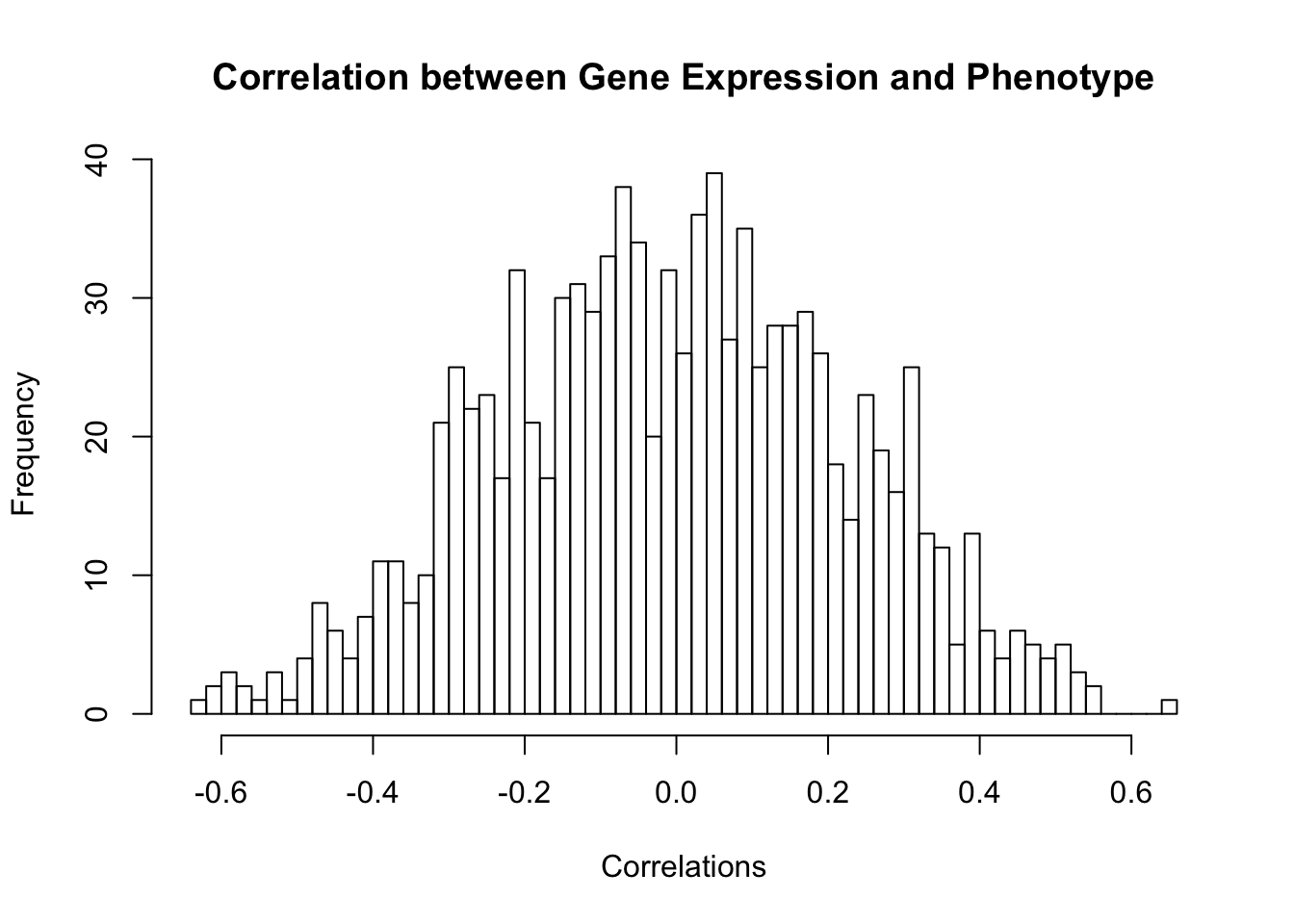We start by generating 20 random normals to represent the phenotypic scores of 20 samples and saving them in a variable called pheno.

nsamp=20
pheno=rnorm(nsamp)

Then we generate 1000 gene expressions. Each has a value for each of the 20 samples. Typically gene expression values are stored in a matrix with genes in the rows and samples in the columns. This matrix is therefore 1000 by 20.

ngene=1000
genes=matrix(rnorm(nsamp*ngene),ncol=nsamp)

Next we compute the correlation between the phenotypic score and gene expression. The R cor command computes the correlation between the columns of two matrices.

Since the genes are in the rows, we need to transpose the gene expression matrix to compute the correlations.

cors=cor(t(genes),pheno)

To visualize the correlations, we draw a histogram. Since we have 1000 correlations, we can have quite a few bins - I selected 50 but R adjusts this based on some algorithm.

hist(cors,nclass=50,xlab="Correlations",main="Correlation between Gene Expression and Phenotype")Next we select the 10 genes with the largest absolute correlation. These are the genes the are individually most predictive of phenotypic score (in this data set).

nsel=10
selgenes=order(cors,decreasing=FALSE)[1:nsel]
sigGenes=genes[selgenes,]

Finally, we do the linear regression on the fitted genes and compute the $$R^2$$.

regout=lm(pheno~t(sigGenes))
R2=var(fitted(regout))/var(pheno)
anova(regout)
## Analysis of Variance Table
##
## Response: pheno
##             Df  Sum Sq Mean Sq F value    Pr(>F)
## t(sigGenes) 10 19.6963 1.96963  13.757 0.0002724 ***
## Residuals    9  1.2886 0.14317
## ---
## Signif. codes:  0 '***' 0.001 '**' 0.01 '*' 0.05 '.' 0.1 ' ' 1
R2
##  0.9385954